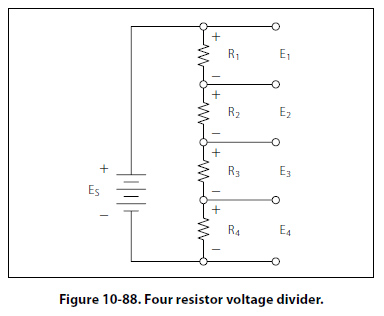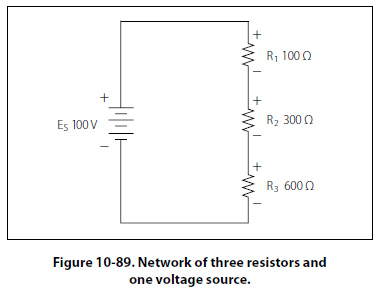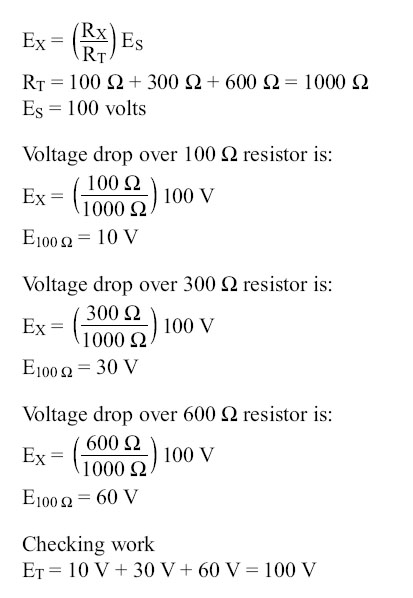Determining the Voltage Divider Formula Figure 10-88 shows the example network of four resistors and a voltage source. With a few simple calculations, a formula for determining the voltage divisions in a series circuit can be determined.The voltage drop across any particular resistor shall be called EX, where the subscript x is the value of a particular resistor (1, 2, 3, or 4). Using Ohm’s law, the voltage drop across any resistor can be determined. Ohm’s law: EX = I (RX) As seen earlier in the text, the current is equal to the source voltage divided by the total resistance of the series circuit. Current: I = ES/RT The current equation can now be substituted into the equation for Ohm’s law. Substitute: Ex =(Es/Rt)(Rx) Algebraic rearrange: Ex =(Rx/Rt)(Es) This equation is the general voltage divider formula. The explanation of this formula is that the voltage drop across any resistor or combination of resistors in a series circuit is equal to the ratio of the resistance value to the total resistance, divided by the value of the source voltage. Figure 10-89 illustrates this with a network of three resistors and one voltage source.©AvStop Online Magazine                                                                                                                                                      Contact Us              Return To Books